[弱平稳过程]  如果随机过程{x (t),tÎT}满足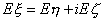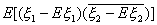[相关函数的谱分解]  如果函数R(τ)是某一均方连续平稳过{x (t),<t<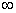}的相关函数，那末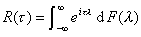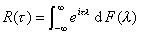{x (t),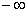<t<}是实值平稳过程时，相关函数R(τ)可以表示成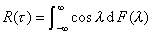(当谱密度存在时)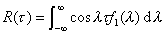(k=0,±1,L)

F(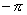)=0,     F(p)=R(0)

[遍历性定理]

1°  如果{x (t),-<t<}是均方连续的平稳过程，那末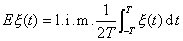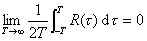2°  如果{x n, n=0,±1,L}是平稳序列，那末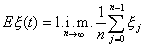3°  如果{x (t),-<t<}是均值为零的均方连续的平稳过程，又对取定的常数  t >0,也是均方连续的平稳过程，记其相关函数为Rt (u),那末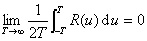4°  如果{n=0,±1,L}是均值为零的平稳序列，又对取定的整数m,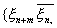n=0,±1,L}也是平稳序列，记其相关函数为Rm(k),那末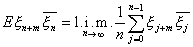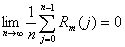[平稳过程的谱展式]  如果{x (t),-<t<}是均值为零的均方连续平稳过程，那末有ii当区间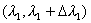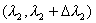不相重叠时(即Z(l )是具正交增量的过程)

iii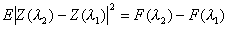(F(l )是谱函数)

Z(l )称为x (t)的随机谱函数，x (t)的积分表示式称为x (t)的谱展式。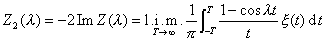ii当区间不相重叠时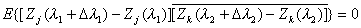(j,k=1,2)

iii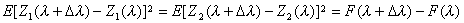(F(l )谱函数)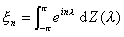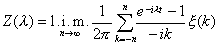-p λp